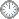# “执行定理”的证明(b+)

This is an in-mail from TYUST.
新入の者--> What is going on ? (redirected)new

(接前: 30 28 25) “执行定理” (Th1.6)的证明(b+).
.

---- 由 A 的条件推出 X 属于有界族.
---- 则 X 的本征像配对 (W, Γ) 属于有界族.
---- 对其“调整版”(W, δΓ) 做MMP 得 X'.
(得到 X' 可看做 “站点”).
---- 4 个 Cartier 推出 (X, 0) eps-lc.
---- 写出锻公式: Kw + δΓ =φ*Kx + E.
(得到锻公式可看做“站点”, E 为代表).
---- 用负性引理联系两个站点(E 在 X' 之上压缩).
---- 进而 X' --> X 是 X 的Q-因式化.
.

---- 第一次靠 X 系有界族驱动.
---- 第二次靠MMP 驱动.
.

---- 原作的头脑中想的该是 X 的Q-因式化.(?)
---- 由此向前反推, 走通后做了正向叙述.(?)
(Q-factorialisation 和 负性引理 待考?)
.

.

.
If X is not Q-factorial, then X' is not unique but since (W, Γ) belongs to a bounded family of pairs, we can choose X' so that it belogs to a bounded family of varieties depending only on d, r.
---- 此处只考虑了 X 不是 Q-factorial的情况.
---- 由 (W, Γ) 属于有界族, 可使 X' 属于有界族.
(有界族属性在一定条件下可以传递)
.
5
|    |
para2.8
|    |
11  12
.

---- g: 假定 X 非Q-factorial.
---- 11: X' 不唯一.
---- 12: 选 X' 属于有界族.
.
Let Kx' + B' and A' be the pullbacks of Kx + B and A.
---- 将主要对象 B 和 A 转到 X' 空间.
.
B   A
|    |
para2.9
|    |
B'  A'
.

.
We can choose a very ample divisor H' on X' with bounded H' such that H' - A' is ample.
---- 此句可能源于 X' 属于有界族.
(推测: 有界族 <=?=> 有界多重性)
.
12
|
para2.10
|     |
13  14
.

---- 13: H' ~ very ample (on X'), H' bounded.
(不妨将条件 b 称作“有界多重性”).
---- 14: H' - A' ample.(为下句准备, 但来由待考?)
.

.
This ensures H' - B' is ample too as A' - B' is nef.
---- (H' - A') ample + (A' - B') nef = H' - B' ample.
(ample + nef = ample ?
---- 条件中有 A - B ample, 但此处是 A' - B' nef.
.
c  14
?|    |
para2.11
|
15
.

---- 15: H' - B' ample.
.
Now lct(X, B, |A|R) = lct(X', B', |A'|R lct(X', B', |H'|R).
---- 等式可能源于两套对象间的等价关系.
---- H' - A' ample ==> A' 比 H' “小” ==> 不等式.
.
h   14
?|     |
para2.12
|     |
16  17
.

----   h两套对象等价.
---- 16: lct 等式.
---- 17: lct 不等式.
.

.
Therefore, replacing X, B, A with X', B', H', and replacing r accordingly, we can assume X is Q-factorial.
---- 之前的落点“10” 意味着 X' 系 Q-factorial.(?)
---- 若不替换, 直接在X'空间向5.9靠拢不行吗?
---- 注意, 替换后用了“we can assume” 句型, 其中必有缘故...(?)
.

符号大全上下标.|| 常用：↑↓ π ΓΔΛΘΩμφΣ∈  ∪ ∩ ⊆ ⊇ ⊂ ⊃ ≤ ≥ ⌊ ⌋ ⌈ ⌉ ≠ ≡ ⁻⁰ ¹ ² ³ ᵈ ₀ ₁ ₂ ₃ ᵢ .

#### Glossary(AG)

*

Introduction
....
....
11/9
...
Proposition 5.5 11/5

http://blog.sciencenet.cn/blog-315774-1196014.html

## 全部精选博文导读

GMT+8, 2020-9-30 16:19